# 06-240/Classnotes For Thursday November 9

## Review of Last Class

 Problem. Find the rank (the dimension of the image) of a linear transformation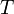$T$ whose matrix representation is the matrix A shown on the right.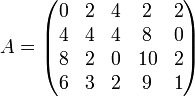$A=\begin{pmatrix}0&2&4&2&2\\4&4&4&8&0\\8&2&0&10&2\\6&3&2&9&1\end{pmatrix}$.
 Theorem 1. If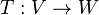$T:V\to W$ is a linear transformation and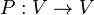$P:V\to V$ and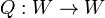$Q:W\to W$ are invertible linear transformations, then the rank of$T$ is the same as the rank of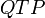$QTP$. Proof. Owed. Theorem 2. The following row/column operations can be applied to a matrix$A$ by multiplying it on the left/right (respectively) by certain invertible "elementary matrices": Swap two rows/columns Multiply a row/column by a nonzero scalar. Add a multiple of one row/column to another row/column. Proof. Semi-owed.

Solution of the problem. using these (invertible!) row/column operations we aim to bring$A$ to look as close as possible to an identity matrix, hoping it will be easy to determine the rank of the matrix we get at the end:

 Do Get Do Get 1. Bring a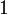$1$ to the upper left corner by swapping the first two rows and multiplying the first row (after the swap) by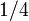$1/4$.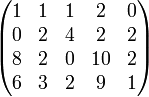$\begin{pmatrix}1&1&1&2&0\\0&2&4&2&2\\8&2&0&10&2\\6&3&2&9&1\end{pmatrix}$ 2. Add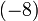$(-8)$ times the first row to the third row, in order to cancel the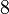$8$ in position 3-1.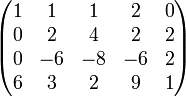$\begin{pmatrix}1&1&1&2&0\\0&2&4&2&2\\0&-6&-8&-6&2\\6&3&2&9&1\end{pmatrix}$ 3. Likewise add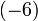$(-6)$ times the first row to the fourth row, in order to cancel the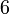$6$ in position 4-1.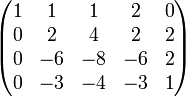$\begin{pmatrix}1&1&1&2&0\\0&2&4&2&2\\0&-6&-8&-6&2\\0&-3&-4&-3&1\end{pmatrix}$ 4. With similar column operations (you need three of those) cancel all the entries in the first row (except, of course, the first, which is used in the canceling).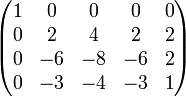$\begin{pmatrix}1&0&0&0&0\\0&2&4&2&2\\0&-6&-8&-6&2\\0&-3&-4&-3&1\end{pmatrix}$ 5. Turn the 2-2 entry to a$1$ by multiplying the second row by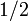$1/2$.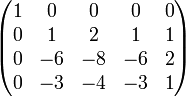$\begin{pmatrix}1&0&0&0&0\\0&1&2&1&1\\0&-6&-8&-6&2\\0&-3&-4&-3&1\end{pmatrix}$ 6. Using two row operations "clean" the second column; that is, cancel all entries in it other than the "pivot"$1$ at position 2-2.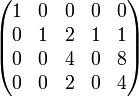$\begin{pmatrix}1&0&0&0&0\\0&1&2&1&1\\0&0&4&0&8\\0&0&2&0&4\end{pmatrix}$ 7. Using three column operations clean the second row except the pivot.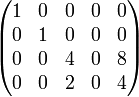$\begin{pmatrix}1&0&0&0&0\\0&1&0&0&0\\0&0&4&0&8\\0&0&2&0&4\end{pmatrix}$ 8. Clean up the row and the column of the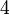$4$ in position 3-3 by first multiplying the third row by$1/4$ and then performing the appropriate row and column transformations. Notice that by pure luck, the$4$ at position 4-5 of the matrix gets killed in action.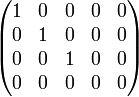$\begin{pmatrix}1&0&0&0&0\\0&1&0&0&0\\0&0&1&0&0\\0&0&0&0&0\end{pmatrix}$

But the matrix we now have represents a linear transformation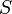$S$ satisfying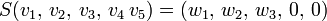$S(v_1,\,v_2,\,v_3,\,v_4\,v_5)=(w_1,\,w_2,\,w_3,\,0,\,0)$ for some bases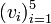$(v_i)_{i=1}^5$ of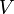$V$ and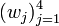$(w_j)_{j=1}^4$ of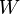$W$. Thus the image (range) of$S$ is spanned by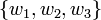$\{w_1,w_2,w_3\}$, and as these are independent, they form a basis of the image. Thus the rank of$S$ is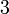$3$. Going backward through the "matrix reduction" process above and repeatedly using theorems 1 and 2, we find that the rank of$T$ must also be$3$.